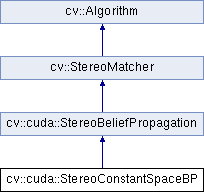OpenCV  3.1.0 Open Source Computer Vision
cv::cuda::StereoConstantSpaceBP Class Referenceabstract

Class computing stereo correspondence using the constant space belief propagation algorithm. : More...

#include "cudastereo.hpp"

Inheritance diagram for cv::cuda::StereoConstantSpaceBP:## Public Member Functions

virtual int getNrPlane () const =0
number of active disparity on the first level More...

virtual bool getUseLocalInitDataCost () const =0

virtual void setNrPlane (int nr_plane)=0

virtual void setUseLocalInitDataCost (bool use_local_init_data_cost)=0Public Member Functions inherited from cv::cuda::StereoBeliefPropagation
virtual void compute (InputArray left, InputArray right, OutputArray disparity, Stream &stream)=0

virtual void compute (InputArray data, OutputArray disparity, Stream &stream=Stream::Null())=0
Enables the stereo correspondence operator that finds the disparity for the specified data cost. More...

virtual double getDataWeight () const =0
data weight More...

virtual double getDiscSingleJump () const =0
discontinuity single jump More...

virtual double getMaxDataTerm () const =0
truncation of data cost More...

virtual double getMaxDiscTerm () const =0
truncation of discontinuity cost More...

virtual int getMsgType () const =0
type for messages (CV_16SC1 or CV_32FC1) More...

virtual int getNumIters () const =0
number of BP iterations on each level More...

virtual int getNumLevels () const =0
number of levels More...

virtual void setDataWeight (double data_weight)=0

virtual void setDiscSingleJump (double disc_single_jump)=0

virtual void setMaxDataTerm (double max_data_term)=0

virtual void setMaxDiscTerm (double max_disc_term)=0

virtual void setMsgType (int msg_type)=0

virtual void setNumIters (int iters)=0

virtual void setNumLevels (int levels)=0Public Member Functions inherited from cv::StereoMatcher
virtual void compute (InputArray left, InputArray right, OutputArray disparity)=0
Computes disparity map for the specified stereo pair. More...

virtual int getBlockSize () const =0

virtual int getDisp12MaxDiff () const =0

virtual int getMinDisparity () const =0

virtual int getNumDisparities () const =0

virtual int getSpeckleRange () const =0

virtual int getSpeckleWindowSize () const =0

virtual void setBlockSize (int blockSize)=0

virtual void setDisp12MaxDiff (int disp12MaxDiff)=0

virtual void setMinDisparity (int minDisparity)=0

virtual void setNumDisparities (int numDisparities)=0

virtual void setSpeckleRange (int speckleRange)=0

virtual void setSpeckleWindowSize (int speckleWindowSize)=0Public Member Functions inherited from cv::Algorithm
Algorithm ()

virtual ~Algorithm ()

virtual void clear ()
Clears the algorithm state. More...

virtual bool empty () const
Returns true if the Algorithm is empty (e.g. in the very beginning or after unsuccessful read. More...

virtual String getDefaultName () const

virtual void read (const FileNode &fn)
Reads algorithm parameters from a file storage. More...

virtual void save (const String &filename) const

virtual void write (FileStorage &fs) const
Stores algorithm parameters in a file storage. More...

## Static Public Member Functions

static void estimateRecommendedParams (int width, int height, int &ndisp, int &iters, int &levels, int &nr_plane)
Uses a heuristic method to compute parameters (ndisp, iters, levelsand nrplane) for the specified image size (widthand height). More...Static Public Member Functions inherited from cv::cuda::StereoBeliefPropagation
static void estimateRecommendedParams (int width, int height, int &ndisp, int &iters, int &levels)
Uses a heuristic method to compute the recommended parameters ( ndisp, iters and levels ) for the specified image size ( width and height ). More...Static Public Member Functions inherited from cv::Algorithm
template<typename _Tp >
static Ptr< _Tp > load (const String &filename, const String &objname=String())
Loads algorithm from the file. More...

template<typename _Tp >
static Ptr< _Tp > loadFromString (const String &strModel, const String &objname=String())
Loads algorithm from a String. More...

template<typename _Tp >
static Ptr< _Tp > read (const FileNode &fn)
Reads algorithm from the file node. More...Public Types inherited from cv::StereoMatcher
enum  {
DISP_SHIFT = 4,
DISP_SCALE = (1 << DISP_SHIFT)
}

## Detailed Description

Class computing stereo correspondence using the constant space belief propagation algorithm. :

The class implements algorithm described in  . StereoConstantSpaceBP supports both local minimum and global minimum data cost initialization algorithms. For more details, see the paper mentioned above. By default, a local algorithm is used. To enable a global algorithm, set use_local_init_data_cost to false .

StereoConstantSpaceBP uses a truncated linear model for the data cost and discontinuity terms:

$DataCost = data \_ weight \cdot \min ( \lvert I_2-I_1 \rvert , max \_ data \_ term)$

$DiscTerm = \min (disc \_ single \_ jump \cdot \lvert f_1-f_2 \rvert , max \_ disc \_ term)$

For more details, see  .

By default, StereoConstantSpaceBP uses floating-point arithmetics and the CV_32FC1 type for messages. But it can also use fixed-point arithmetics and the CV_16SC1 message type for better performance. To avoid an overflow in this case, the parameters must satisfy the following requirement:

$10 \cdot 2^{levels-1} \cdot max \_ data \_ term < SHRT \_ MAX$

## Member Function Documentation

 static void cv::cuda::StereoConstantSpaceBP::estimateRecommendedParams ( int width, int height, int & ndisp, int & iters, int & levels, int & nr_plane )
static

Uses a heuristic method to compute parameters (ndisp, iters, levelsand nrplane) for the specified image size (widthand height).

 virtual int cv::cuda::StereoConstantSpaceBP::getNrPlane ( ) const
pure virtual

number of active disparity on the first level

 virtual bool cv::cuda::StereoConstantSpaceBP::getUseLocalInitDataCost ( ) const
pure virtual
 virtual void cv::cuda::StereoConstantSpaceBP::setNrPlane ( int nr_plane )
pure virtual
 virtual void cv::cuda::StereoConstantSpaceBP::setUseLocalInitDataCost ( bool use_local_init_data_cost )
pure virtual

The documentation for this class was generated from the following file: